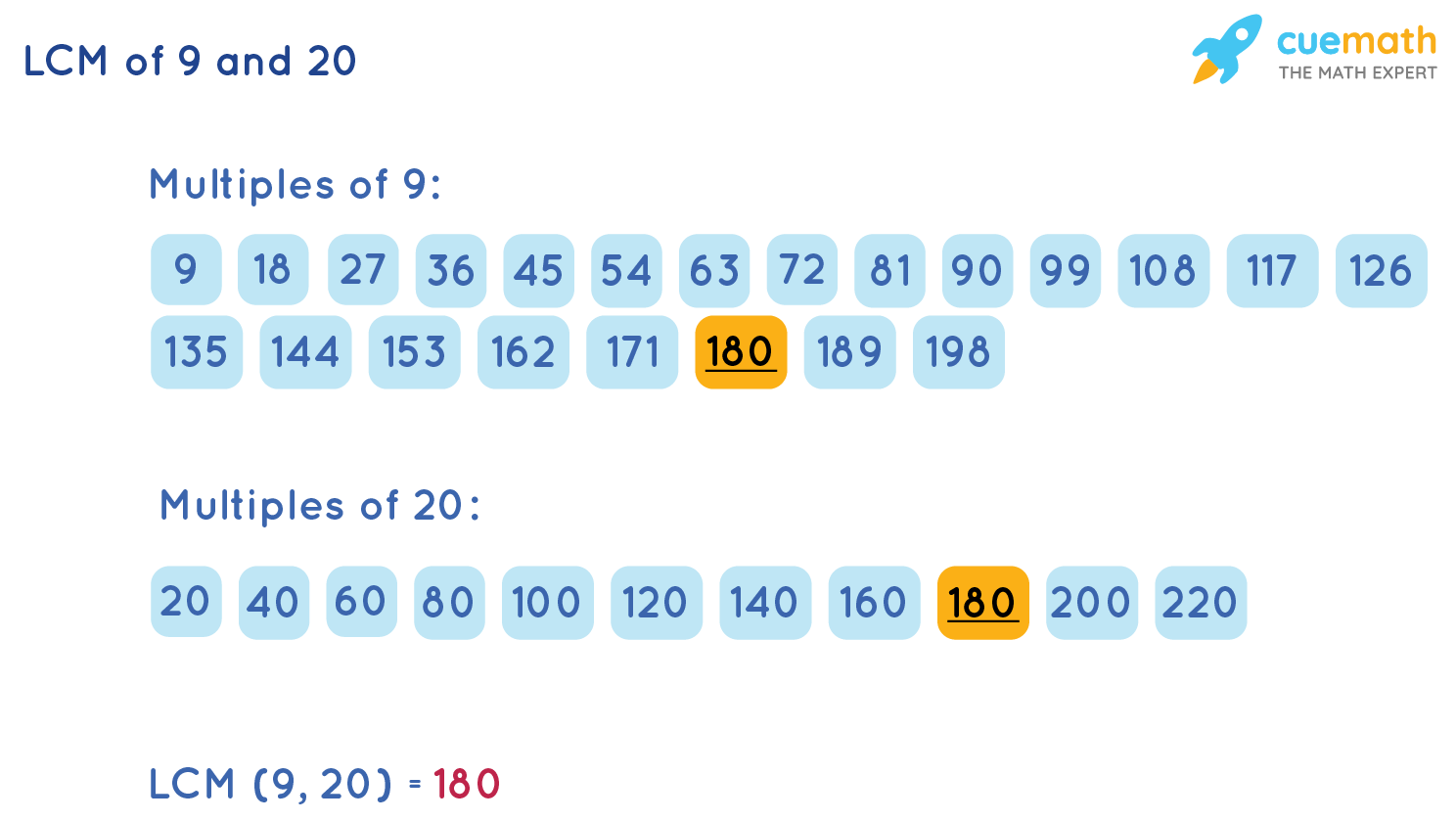# What is the LCM of 9 and 20?

## Question: What is the LCM of 9 and 20?

The LCM (Least Common Multiple) of 9 and 20 is the least number which is exactly divisible by the numbers 9 and 20.

## Answer: LCM of 9 and 20 is 180

Look at the two methods shown below to find the LCM of 9 and 20.

## Explanation:

The two methods that we are using to find the least common multiple of 9 and 20 are shown below.

• LCM of 9 and 20 by Formula
• LCM of 9 and 20 by Listing Method

### Method1: LCM of 9 and 20 by Formula

We will use the formula of LCM (a, b):

LCM (a, b) = (a × b) / HCF (a, b)

HCF (a, b) is the highest common factor of a and b.

Use this formula to find the LCM of 9 and 20.

LCM (9, 20) = (9 × 20) / HCF (9, 20)

= 180 / 1

= 180

Here, 180 is the least common multiple of 9 and 20.

### Method2: LCM of 9 and 20 by Listing Method

Look at the list of multiples of 9 and 20:Observe in this list that 180 is the least common multiple of 9 and 20. So, LCM (9, 20) = 180

By using any of the above methods, the answer to LCM of 9 and 20 is the same.